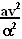## Monday, October 22, 2007

### Irodov Problem 1.91

The radius of curvature of a curve is given by (see here for explanation),The centripetal acceleration experienced by the car as it moves along the curve is given by. The minimum radius of curvature occurs atAt these points the car will experience the maximum centripetal acceleration. The radius of curvature at these point is given byand thus the maximum centripetal acceleration that car will experience as it moves along is given by(note that is becomes the centrifugal force when in the frame of the car).

The maximum friction force that can be provided is mgk and this must provide the ncessary centripetal acceleration at all points in the curve (see 1.90 for an explanation for why since both problems are essentially similar). In other words at the centripetal acceleration experienced by the car must be less than or equal to the maximum force of friction that can be provided. This means,